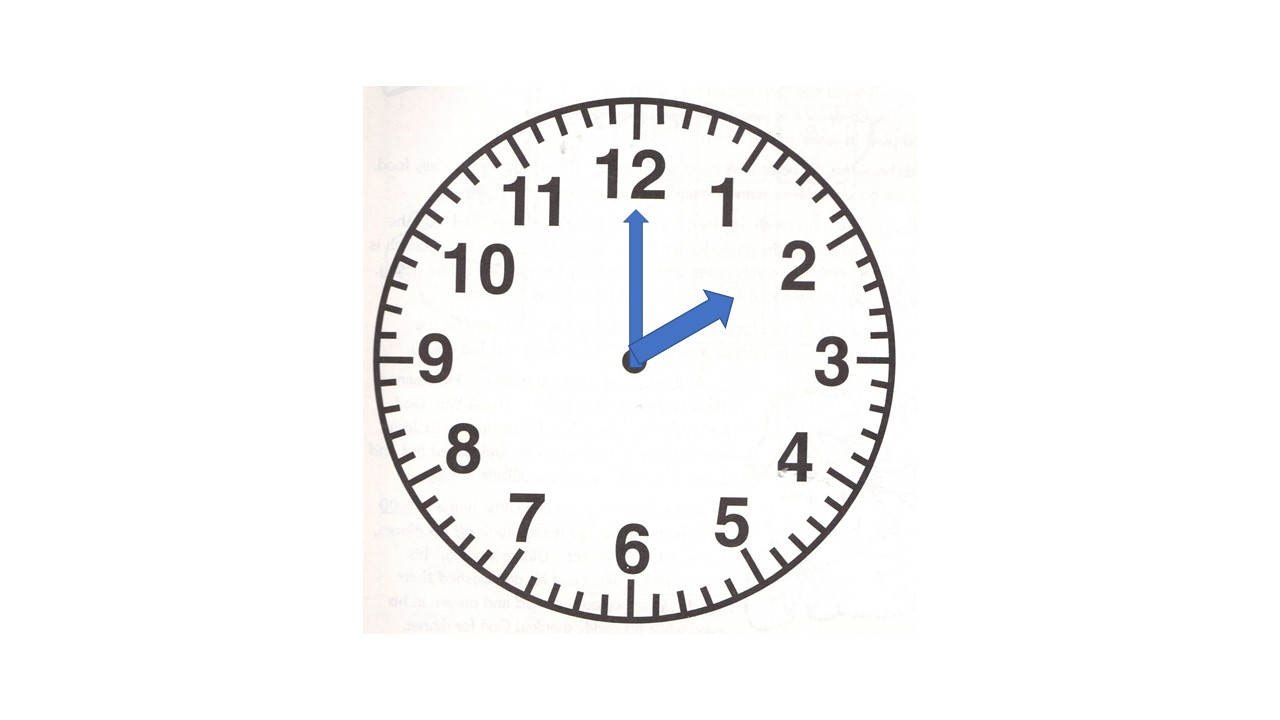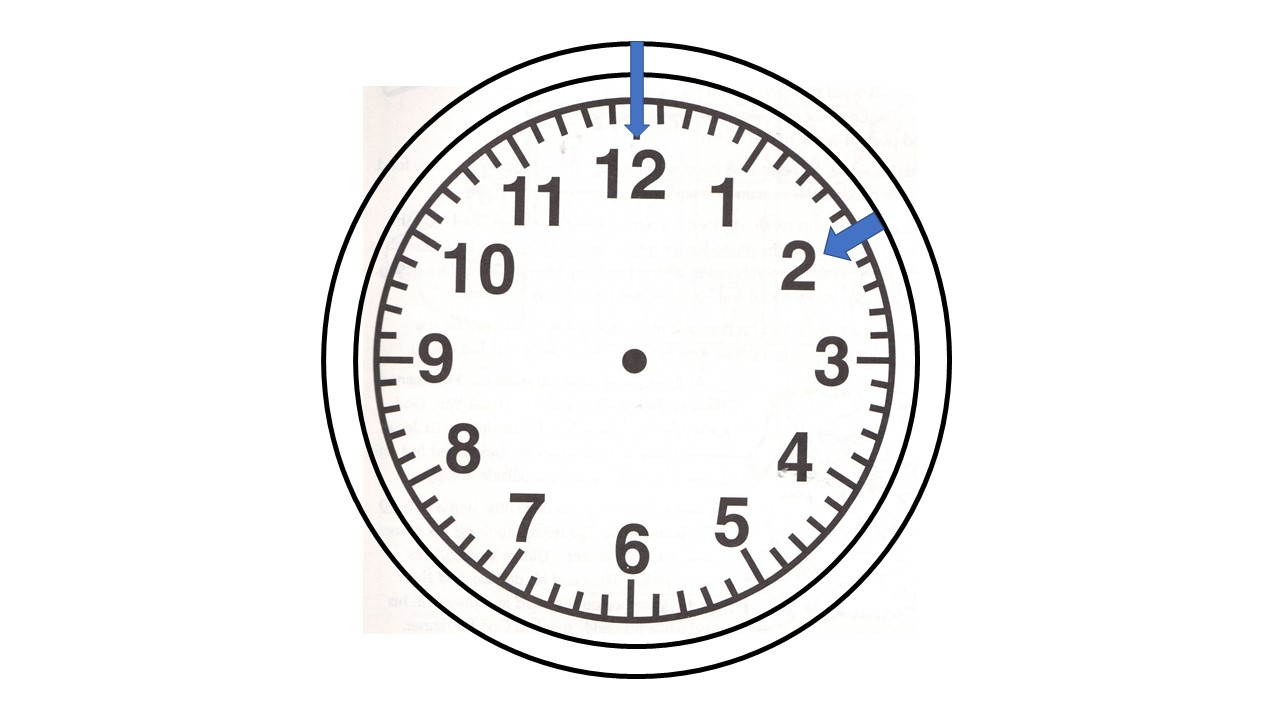# Odologe

An odologe (o’-do-loje) is a continuous, independent measurement of length. That is, it measures an ever-increasing length, which is the placepoint, similar to how a clock shows the timepoint. The term is a combination of (h)odo(s) (as in path) and loge (as in horologe, a clock). An odologe is an odometer that runs constantly at a standard rate. For practical purposes, an odologe may be like a clock that measures the circumference rather than the angle.

What does an odologe look like? Start with a sector, which is a geometric figure fixed to the center of a circle that sweeps out an angle and a curved edge:

In an analogue clock, each hand sweeps out a sector from the vertical 12:In the clock above, the hour hand sweeps out an angle of 2/12 of a circle, or 60 degrees, and the minute hand sweeps out a zero-degree angle. These angles are interpreted as a proportion of the 12-hour day and 60-minute hour, respectively.

The curved edge of a sector is an arc, which is a portion of the circumference. In the image of a odologe below, the hands point in from a circumferential band and measure the arc of the sector indicated, which is interpreted as a proportion of the length of the circumference:Here the hour hand indicates an angle of 2/12 of a circle, or 60 degrees, and the minute hand indicates a zero degree angle. These angles are interpreted as a proportion of the unit circumference and a proportion of 1/60th of the unit circumference, respectively. The circumferential bands reinforce that it is the arc length being measured.

The length of the circumference must be a known or nominal value, such as one foot or one metre. A foot is convenient since it is divided into 12 inches. The length is accumulated when the hands finish their cycles. The rates of motion are standardized and may equal those of a corresponding clock.

A clock and an odologe are similar, except that a clock measures time and an odologe measures length. As the current position of a clock shows the current point in time, or timepoint, so the current position of an odologe shows the current placepoint.